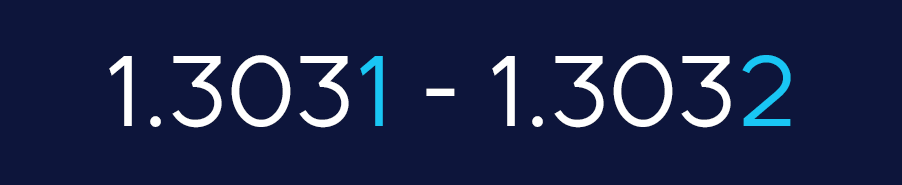# Understanding Pips

### What is a pip?

If you have done any research on forex before or if you have spent some time looking over the markets already then you will surely have come across the term “pip” (sometimes referred to as a “point”).

Note: It’s vital that you understand what a pip is as this will be the fundamental unit that you measure your forex trades in. When we make a trade, we normally target a predetermined number of pips for our entry points and stop losses.

A pip (percentage in point) is the unit of measurement that we use to express the change in value between the currencies in our currency pair.

To be exact, a pip is a standardised unit and is the smallest amount that any currency pair quote can change. Because of this, a pip is usually the last decimal place in a currency pair.

As a rule of thumb, most of the currency pairs in the forex market are quoted to four decimal places. In this instance, the fourth decimal place is the pip, as shown below.In fact, all of the majors are quoted to four decimal places, except for when the Japanese yen is the quote currency. In those instances, there are only two decimal places, and yeah, you guessed it, the last decimal place is the pip, as shown below.### Pips in action

Let’s take a look at some examples of market movements in terms of their pip value.

Example: one pip move

If the value of the GBP rises against the dollar by one pip then we would see a move like this.In this example, the value of GBP has risen by 1 pip against USD. If we were longing on this move, we would have made a 1 pip profit.

Example: 100 pip move

If the value of the GBP rises against the dollar by 100 pips then we would see a movie like this.Average pip movement in the market

On average, forex markets usually move anywhere between 80-100 pips per day. Of course, this differs between each market but that is a reasonable average to draw from.

Now, this may not seem like much, and on the grand scheme of things it isn’t, but when we include the use of leverage and margin trading, we can profit quite significantly from these kinds of moves in the market. More on that later in this unit.

### Pipettes

If you go on to your brokerage and see that the Yen is trading with three decimal places or if you see GBP/USD trading to 5 decimal places, don’t worry.

As a rule of thumb, if there are five decimal places displayed, then the fourth decimal is the pip. If there are three decimal places, then the second is pip.

In these examples, the 5th and 3rd pips would be what we refer to as pipettes and are not particularly important in terms of our trading strategies.

error: